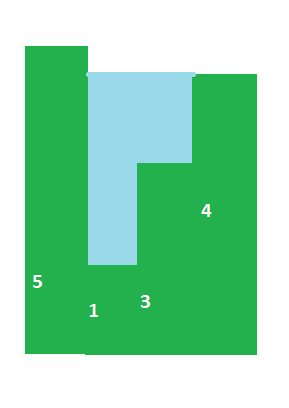Categories

# Maximum Water Capacity in a HistogramCalculate the maximum amount of water that can held within a bar graph without overflow.
The amount of water that can be held at any point is the difference of the minimum of the maximum of left bars and maximum of right bars and the height of that point.
If we don’t precompute the maximum values on left and right for every index, the time complexity becomes O(n^2). So we go with the precompute option.

Time Complexity O(n)
Space Complexity O(n)

Code

```package algos;

import java.lang.Math;

public class maxWater {
public static void main(String[] args) {
int[] input = { 5, 1, 3, 4 };
int len = input.length;
int[] left = new int[len];
int[] right = new int[len];
int rightMax = input[len - 1], leftMax = input;
for (int i = 1; i < len - 1; i++) {
leftMax = Math.max(leftMax, input[i]);
left[i] = leftMax;
rightMax = Math.max(rightMax, input[len - 1 - i]);
right[len - 1 - i] = rightMax;
}
int count = 0;
for (int i = 1; i < len - 1; i++) {
count += Math.min(left[i], right[i]) - input[i];
}
System.out.println(count);
}
}
```

Output

`4`